Trial ends in
JoVE Science Education
Mechanical Engineering

A subscription to JoVE is required to view this content.

Hot Wire Anemometry
##### Science Education (Mechanical Engineering)
Buoyancy and Drag on Immersed Bodies
##### Science Education (Mechanical Engineering)
Jet Impinging on an Inclined Plate

# Hot Wire Anemometry

### Overview

Source: Ricardo Mejia-Alvarez and Hussam Hikmat Jabbar, Department of Mechanical Engineering, Michigan State University, East Lansing, MI

Hot-wire anemometers have a very short time-response, which makes them ideal to measure rapidly fluctuating phenomena such as turbulent flows. The purpose of this experiment is to demonstrate the use of hot-wire anemometry.

### Principles

The hot-wire anemometer

A hot-wire anemometer is a device used to measure flow velocity based on the heat dissipated from a very thin electrically-heated wire. The heat generated by an electric wire,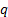, is given by the relationship:(1)

Where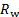denotes the wire's electric resistance andthe electric current flowing through the wire. The electric resistance depends on the wire's temperature according to the following relationship: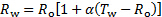(2)

Whereis the wire resistance at the reference temperature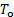and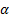is a constant that depends on the material of the wire. While equation (1) expresses the heat generated by the electric current, the heat dissipated by the flow, follows King's law :(3)

Here,,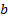, and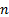are calibration constants and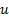is the flow velocity around the wire. The value of n depends on the Reynolds number, and it has already been found that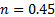is satisfactory for the range of Reynolds numbers achievable in this particular experiment. To obtain a relationship between temperature, electrical current, and velocity, we combine equations (1) and (3):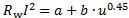(4)

Here, the temperature dependence enters through the electrical resistance (equation (2)). The measurement strategy that we will use in the current experiment is to maintain the temperature (and hence the resistance) of the wire constant. From equation (4), it is clear that if the electrical resistance is constant, the current needs to fluctuate to follow the tendency of the velocity. In other words, cooling rate changes with flow velocity, and that would change the wire's temperature unless the current is changed to compensate. Obviously, it is necessary to have a quick responding electric system to measure a rapidly varying velocity signal. This is achieved with a Wheatstone bridge like the one shown in Figure 1(A). From the figure, the hot-wire is one of the four resistors in the circuit. Figure 1(B) shows its physical configuration, which is a very thin wire set between two prongs (a 5 μm tungsten wire for the current experiment). The control resistor,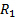, from Figure 1(A) is adjusted initially to produce a zero bridge-voltage,, for the desired baseline temperature (ergo electrical resistance) of the hot-wire. When in operation, the bridge-voltage is used as a feedback signal to increase or decrease the current to the wire in order to maintain the hot-wire at a constant temperature. On the other hand,, is amplified to achieve an easier-to-read voltage scale,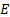. This voltage is related to the current through Ohm's law:(5)

Hence, equation (4) can be expressed in terms of the voltage as: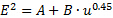(6)

With the calibrations constants now defined as: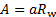and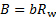. The main purpose of this experiment is to find the value of these calibration constants. To this end, the hot-wire probe will be set in a reference flow system. This flow system will be used to issue several flows with known velocities. Then, the calibration constants will be found using a least-squares regression.

As shown in the schematic of Figure 2, the reference flow to use herein is the vena contracta of a free jet. The mean velocity at the vena contracta is well characterized by the following equation [3, 4, 5]: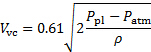(7)

Here, the constant 0.61 is the discharge coefficient of the jet,is the pressure inside the plenum, and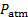is the atmospheric pressure. The position of the vena contracta is well defined by the relationship: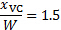(8)

Whereis the distance from the jet exit along its centerline and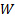is the width of the slit from where the jet is issued. This is the location where the hot-wire anemometer will be located for its calibration. Figures 3 and 4 show the flow system used herein. In this system, a fan pressurizes a plenum that has two exits, a slit to produce the jet, and a stack to divert the flow. As the flow through the stack is restricted with orifice plates (see Figure 4 for reference), the flow rate of the jet increases. This setup will help us producing a scatter plot of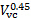and the voltagemeasured at the Wheatstone bridge.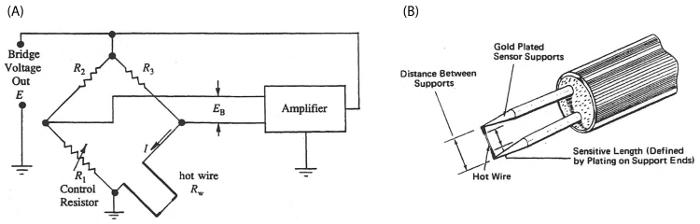Figure 1. Schematic of the planar jet showing: the vena contracta and the diagram of connections. Please click here to view a larger version of this figure.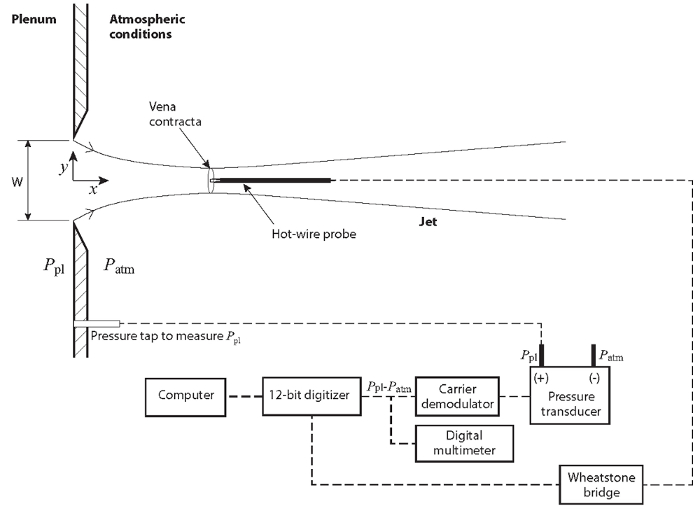Figure 2. Experimental setup. (A): flow facility; the plenum is pressurized by means of a centrifugal fan. (B): slit for issuing the planar jet. (C): traversing system to change the position of the anemometer along the jet. Please click here to view a larger version of this figure.

### Procedure

1. Measure the width of the slit, W, and record this value in table 1.
2. Verify that the data acquisition system follows the schematic in Figure 2.
3. Connect the positive port of the pressure transducer (see Figure 2 for reference) to the plenum pressure tap ().
4. Leave the negative port of the pressure transducer open to the atmosphere. Hence, the reading of this transducer will be directly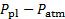; as required by equation (7).
5. Start the program for hot-wire calibration. Set the sample rate at 100 Hz for a total of 1000 samples (i.e. 10s of data).
6. Make sure that channel 0 in the data acquisition system corresponds to the voltage of the hot-wire anemometer.
7. In the field corresponding to channel 0, select the value of the constantto 0.45.
8. Set the hot-wire anemometer at the position of the vena contracta (on the centerline, at x = 1.5 W).
9. Make sure that channel 1 in the data acquisition system corresponds to the signal of the pressure transducer.
10. Enter the values of local air density (typically 1.2 kg/m3 for average local conditions) and conversion constant from volts to pressure (76.75 Pa/V) in the fields corresponding to the pressure transducer. Record these values in table 1. With this, the data acquisition system will report the data directly in velocity in m/s according to equation (7).
11. Cover the stack completely to establish the condition for maximum velocity at the jet.
12. Turn on the flow facility.
13. Acquire a dataset.
14. Change the stack plate for one with a lower restriction (larger diameter)
15. Acquire a dataset.
16. Repeat steps 1.15 and 1.16 for a total of at least four times. Make sure that the last repetition is carried out with the stack fully unrestricted (lowest jet velocity).
17. The data acquisition program will carry out the least-squares calculation and report the calibration constants automatically. Record these values on table 1.

Table 1. Basic parameters for experimental study.

 Parameter Value Slit width (W) 19.05 (mm) Air density (r) 1.2 (kg/m3) Transducer calibration constant (m_p) 76.75 (Pa/V) Calibration constant A 5.40369 (V2) Calibration constant B 2.30234 (V2 s0.65m-0.65)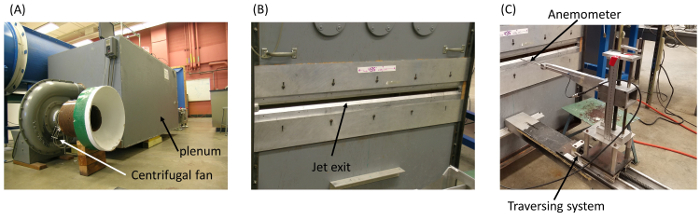Figure 3. Hot-wire anemometer circuitry. (A): Wheatstone bridge circuit to ensure constant temperature in the hot-wire. (B): detail of the structure of a hot-wire. Please click here to view a larger version of this figure.Figure 4. Flow control in the flow system. The stack on top of the plenum serves the purpose of diverting flow from the jet slit allowing to control the jet's exit velocity. Please click here to view a larger version of this figure.

Hot wire anemometry is a common experimental technique for measuring fluid velocity during flow tests. Velocity is often an important parameter of the flow to characterize. But as with many flow properties, it is challenging to measure without impacting the experiment. Measurement of turbulent flows adds an additional complication, since the velocity can fluctuate rapidly in this regime. Hot wire anemometers are a successful diagnostic because they can be made very small so as to minimize their impact on the measured flow. And they have a fast enough time response to resolve rapid velocity fluctuations in the flow. This video will illustrate how a hot wire anemometer works in the constant temperature configuration. And then show how these devices are calibrated in preparation for their use in flow experiments.

In its simplest form, a hot wire anemometer consists of a short resistive wire suspended between two supports and placed in the flow of interest. The wire is electrically heated to a temperature well above the ambient fluid temperature such that the heat generated counteracts the heat lost from convective cooling. Since the convective cooling rate depends on the velocity of the fluid, a relation between the electrical power into the anemometer and the flow velocity can be defined. Joule heating of the wire is the product of the wire resistance and the square of the electric current. For most materials, the resistance is temperature dependent and is well approximated by a linear relationships, where the constant alpha is specified by the wire material. The convective cooling can be modeled using King's Law. In this equation A and B are constants to be determined during calibration. And the exponent of the velocity N is typically around 0.45. At steady state, heating and cooling are balanced. But determining the instantaneous input power depends on knowing both the resistance and current simultaneously. One strategy known as Constant-Temperature Anemometry is to supply only enough current to keep the temperature and hence the resistance of the wire constant. This is accomplished using a Wheatstone Bridge with the hot wire serving as one leg of the bridge circuit. The bridge can be thought of as two voltage dividers in parallel, and the difference in their outputs is the bridge voltage. If the ratios are one to R2 and RW to R3 are equal, the bridge is balanced and the bridge voltage is zero. Otherwise, the bridge voltage is amplified and used as a feedback signal. Current will then flow through the hot wire, heating it until the bridge is brought into balance. The baseline temperature of the hot wire can be set by adjusting the control resister R1. Ohm's Law can be used to relate the current flowing through the hot wire to the bridge voltage. Now, since our W is held constant, the resistance terms can be absorbed into the calibration constants. And the velocity is specified as a function of the bridge voltage. The calibration constants are determined by placing the anemometer in a reference flow system. Measurements must be taken at several known flow velocities so that a least squares regression can be performed. The vena contracta of the free jet is a useful reference flow because the mean velocity is well characterized by the fluid density and pressure differential. Now that you understand how the hot wire anemometer works, let's see how the device can be calibrated experimentally using the vena contracta of a free jet.

Before you begin setting up, familiarize yourself with the layout and safety procedures of the facility. The flow system consists of a plenum pressurized by a centrifugal fan. A free jet forms from the slit on the side of the plenum and the stack on the top allows the plenum pressure to be adjusted by swapping restricting orifice plates. Now setup the data acquisition system as shown in the diagram in the text. Connect the plenum pressure tab to the positive port of the pressure transducer and leave the negative port open to the atmosphere. Set channel zero on the data acquisition system to the bridge voltage of the anemometer and channel one to the pressure transducer. In the data acquisition software, set the hot wire channel to zero and the pressure transducer channel to one. Finally, set the constant N to 0.45 and the sampling rate to 100 Hertz and the total samples to 1000. Enter the values for the local air density in the transducer calibration constant in the appropriate fields so that the data acquisition system reports the results in meters per second. Use a calibrated spaced to set the slit width to 19.05 millimeters or three quarters of an inch. And then affix the hot wire anemometer in the flow at the position of the vena contracta which is at a distance of 1.5 times the slit width from the opening. Locate the anemometer and the mid plane of the jet by traversing the probe in the cross stream direction until the signal on the oscilloscope shows minimal fluctuations. Now cover the stack completely to establish the maximum jet velocity and then turn on the flow facility. Use the software to record a data point. After the measurement is complete, exchange the orifice plate for one with the smallest opening. Take another measurement with the new orifice plate. Continue exchanging orifice plates and taking measurements until you have collected at least six data points including one with the stack fully unrestricted. Recover the calibration constants from the data acquisition software.

Once you have finished collecting data, you will have voltage measurements from the bridge circuits corresponding to a range of different flow velocities. Plot the voltage squared as a function of velocity raised to the power 0.45. And then perform a least squares linear fit to the data. The slope and intercept from the fit are the calibration constants for this hot wire anemometer. Now that the anemometer has been calibrated it can be used in a different flow setup to measure an unknown velocity.

Hot wire anemometers are frequently used in scientific flow experiments. Hot wire anemometry is extensively used to study boundary layer flows in wind tunnels. Boundary layers are one of the oldest subjects of research in fluid mechanics due to their relevance to technological applications such as aerodynamic design, naval engineering, and power generation among others. To the detriment of all these fields, many effects involving the boundary layer are still incipiently understood. Highly irregular roughness, density and viscosity gradients and compressibility to mention a few. With this in mind, hot wire anemometry is used in laboratory settings to assess boundary layer flows relevant to the above mentioned applications. Using strategies similar to the one demonstrated in the current experiment. Industrial ventilation systems are used to control fumes, particulates, aerosols, combustion products or other contaminants in industrial settings. Commonly, each contaminant will be generated at a different rate. Hence, the exhausting system will require a different flow rate for each contaminant to eliminate them efficiently. This procedure is typically carried out with the help of a hot wire anemometer. Wherein the engineer sets the position of a damper in each line, seeking to make the reading of the anemometer match a pre-estimated velocity value.

You've just watched Jove's introduction to hot wire anemometry. You should now understand how a constant temperature anemometer works, and how to calibrate this device for use in flow experiments. Thanks for watching.

### Results

The experimental measurements are listed in Table 2 and shown in Figure 5. A linear regression of these data produced the following result for equation (6):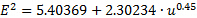(9)

Which can be used to determine the velocity as a function of the voltage:(10)

Table 2. Representative results. Measurements of voltage square and velocity at the vena contracta to the 0.45 power.

 V_VC^0.45 E^2 3.119 12.584 3.919 14.425 4.143 14.946 4.278 15.256 4.465 15.679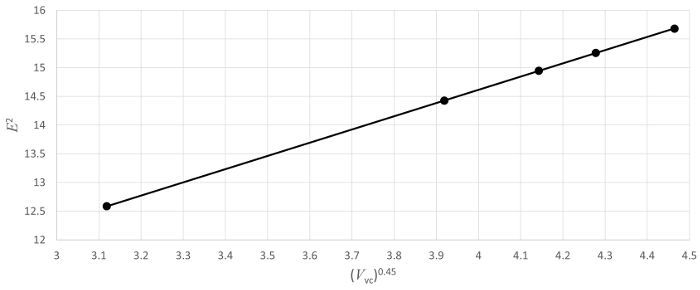Figure 5. Hot-wore anemometer's calibration curve. Please click here to view a larger version of this figure.X